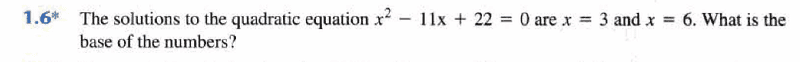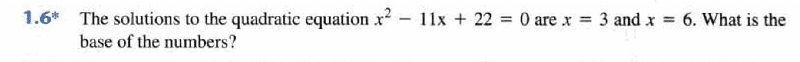# [Help] with Numbering System Digital Circuit

• Engineering
[Help] with Numbering System "Digital Circuit"

Hello Guys ..

iam having an exam on digital circuits and i try to work on some problems about numbering systems

and i found this problems but i couldn't solve it ..

i said that (x-3)(x-6)=x^2-11x+22
then i said that

x^2-9x+18=x^2-11x+22
so 2x=4 and x=2 but i didn't know what to do after that ..so please help meThx Guys !!

berkeman
Mentor
Hello Guys ..

iam having an exam on digital circuits and i try to work on some problems about numbering systems

and i found this problems but i couldn't solve it ..

i said that (x-3)(x-6)=x^2-11x+22
then i said that

x^2-9x+18=x^2-11x+22
so 2x=4 and x=2 but i didn't know what to do after that ..so please help meThx Guys !!

If 3 * 6 = 22, what is the base?

And this is for a logic circuits class? Really?

phinds
Gold Member
X=3 and X=6 are not the same solution at the same time, so saying that (x-3)(x-6)=ANYTHING is not helpful.

The solution falls out of Berkeman's approach immediately, but if you want an algebraic (and more complicated) method, try this:

Express the equation you are given as a function of an assumed base B and express everything in the equation as a decimal equivalent and then solve for B. It's trivial, really, whether you start with 3 or start with 6.

And REMEMBER, expressed as a decimal number, "22-base-B" is 2*B + 2 and similarly for the 11.

This was the first chapter of Digital Circuits ..an Introduction for binary and other numbering systems ...this was the first section of the course :D

and if 3 * 6 = 22
then 3 * 6 = 2+2x then base = 8berkeman
Mentor
There are 10 kinds of people in the world...Hahahah ..i think ..it depends on the base we are using , Berkeman